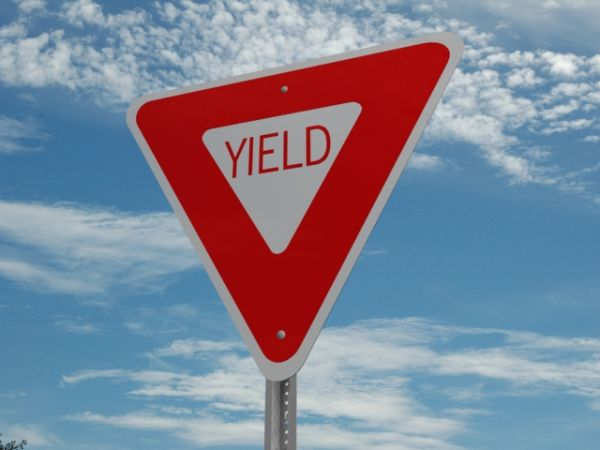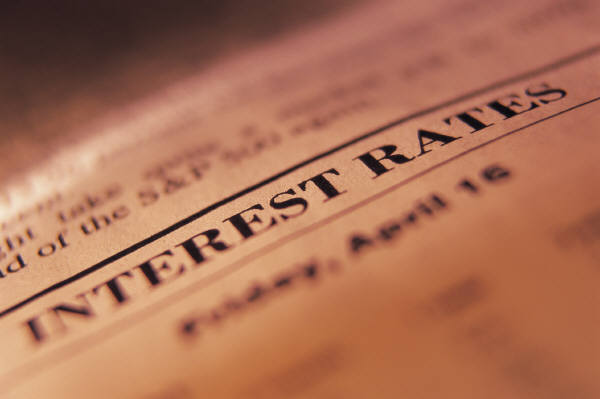# Difference Between Yield and Interest Rate

If you want to make smart investments or calculate your investment profits, it is very important for you to know the difference between yield and interest. People often fail to understand the meaning of these two terms. The simplest way to define yield and interest rate is that the former is the profit of your investment, whereas the latter is why you made the profit.

Interest rate is always stated as a percentage of the principal amount. Whether you are getting or paying the interest rate, it is the percentage of the money above the original amount. For example, if you take out a loan of US\$ 100,000 with a 5% interest rate, you will pay 5% more money than you borrowed.

Yield on the other hand is how much you actually earned or profited from a particular investment. Yield can be stated both as a percentage and exact figures. For example, if you made an investment of US\$ 100,000 with a 3% interest rate for one year, your yield would be about US\$ 3000.

Furthermore, yield can be compounded, but interest rates cannot be. The former is determined using basic mathematical calculations, which involve dividing the interest amount received, by the cost of investment and the time duration, while the latter is calculated by using a simple interest rate formula – Interest = P (Principal amount) x I (Annual interest Rate) x N (number of years).

### Instructions

• 1

Yield:

Investopedia defines Yield as, "The income return on an investment.” This refers to the interest or dividend an investor receives from a security (such as a bond or a stock) and is usually expressed as an annual percentage, based on the investment cost or its face value.

For example if an ABC company pays a quarterly dividend of 50 cents (\$.05) and the stock price is US\$50, then the annual yield would be 4%, calculated by multiplying the dividend amount with number of quarters and dividing the answer by the stock price. If the stock price increases to become US\$100, with the dividend remaining constant, the yield would become 2%.

Image Courtesy: etfmarketpro.com• 2

Interest Rate:

Interest Rate is defined by Investopedia as, "The amount charged, expressed as a percentage of principal, by a lender to a borrower for the use of assets." In order to understand this better, consider this example - You go to a bank to borrow \$100,000 for a new car and the bank quotes you a 5% interest rate on the loan. If you have borrowed the money for one year, then after 12 months you will return \$100,000 plus \$5000 as the interest.

Image Courtesy: lietaer.com•